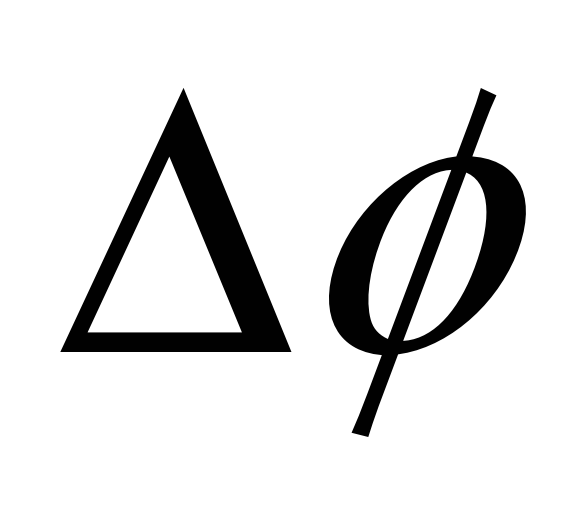# Spacecraft Attitude Determination Using Global Positioning Satellite Signals

 In whichis the baseline length in wavelengths,is the angle between a sightline to the GPS satellite,is the wavelength of the GPS signal,is the fractional phase difference between the two receivers, andis the integer phase difference (number of full wavelengths) between the two receivers (Application of Vectorized Attitude Determination Using Global Positioning Signals). The idea is that if the unit sightline vectors from the spacecraft to at least two GPS satellites in an inertial frame are known, the fractional phase difference between receivers are measured, and the receiver arrangement on the satellite is known, the baseline can essentially be mapped onto the sightline using a transformation matrix from the inertial coordinate frame to the body coordinate frame as shown in figure 2 (Global Positioning System: Theory and Applications Volume II):Download 1.81 Mb.Share with your friends: